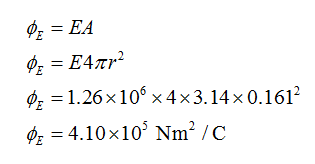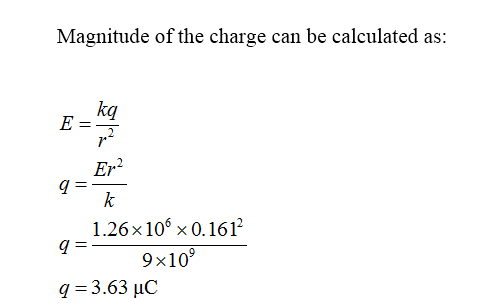# You measure an electric field of 1.26×106 N/C at a distance of 0.161 m from a point charge. There is no other source of electric field in the region other than this point chargeWhat is the electric flux through the surface of a sphere that has this charge at its center and that has radius 0.161 m ?What is the magnitude of the charge?

Question
6 views

You measure an electric field of 1.26×106 N/C at a distance of 0.161 m from a point charge. There is no other source of electric field in the region other than this point charge

What is the electric flux through the surface of a sphere that has this charge at its center and that has radius 0.161 m ?

What is the magnitude of the charge?

check_circle

Step 1

Given:

Electric field = 1.26 X 106 N/C

Distance = 0.161 m

Step 2

Electric flux can be calculated as:Step 3...

### Want to see the full answer?

See Solution

#### Want to see this answer and more?

Solutions are written by subject experts who are available 24/7. Questions are typically answered within 1 hour.*

See Solution
*Response times may vary by subject and question.
Tagged in

### Physics